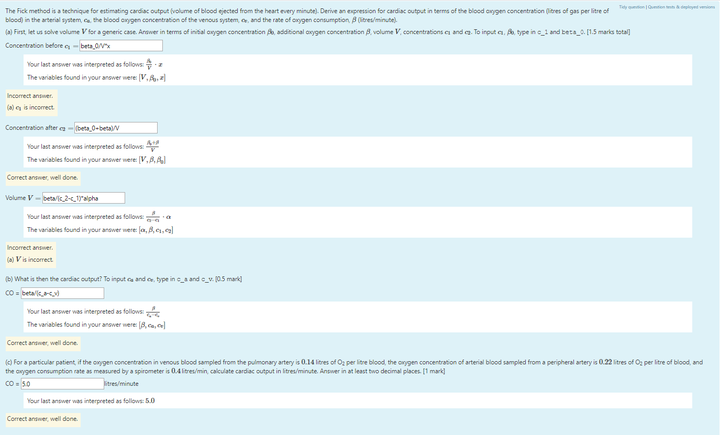# Moodle STACK NotesIn taking teaching assistant roles (i.e., casual academic) in many Biomedical Eng'g courses at UNSW, I had to do countless hours of manual checking. I enjoyed interaction with students but dreaded the marking component. Hence, it is no surprise that I became a fan of automated marking. Moodle is the online MOOC platform used at UNSW and STACK is a symbolic solver plugin. A symbolic solver allows students to input algebraic equations, and automatically check those equations! Below are my personal notes in setting up STACK questions.

### NumDecPlaces

• options: n (number of decimal places)
• Answer must have exactly n decimal places. more or less is wrong
• If the correct answer if 3.456, the checker only checked the first n decimal places, not round it.

### NumDecPlacesWrong

• Tests if the student appears to have the decimal point in the wrong place. For example, 3.141 and 31.41 will be considered the same under this test.
• Checks if the first n numbers are correct. ignore where the decimal points are.

### NumSigFigs

• Liberal and most used test mode in my opinion.
• Option is a list [n,m] then we check the answer has been written with at least n significant figures (can be more), with an accuracy of up to m places. Usually arounded at m places.
• If the options are of the form [n,-1] then the test checks the student has at least n significant figures in the answer, and that numerical accuracy is correct.
• Favorite option: [3,-1]

### Examples

Model Ans.NumDecPlacesNumDecPlacesWrong
1.234567891.2 (n=1)123.456789
0.00123450.0, 1234.5e-6 (n=1)0.0013 (n=2)
1.245e−9124.5e-11 (n=1)1.245e-321 (n=3)

# Multiple Choice Questions (MCQ)

• Not recommend to use MCQ with stack but if you really need it…
• Don't name the figure w/ the correct answer, if the student is smart enough, they can save the image and the uploaded image name will be shown by default. If the answer can be inferred from the image name, it's gonna give them the answer.

### Multiple choice

If want the choices to be diff(p,x), p, and int(p,x) you may declare Tans1 as shown below. Input Tans1 in the model answer and mcq_correct(ta) or mcq_incorrect(ta) in the checker.

Tans1: [[diff(p,x),true],[p,false],[int(p,x),false]]


Input Tans1 in the model answer and the correct answer (e.g., diff(p,x)) in the checker.

# Common Warning Messages

### For cursor drag problems:

• Make sure the cursor/circle (not the text) is inside the box.

### For numerical problems:

• Answer numerically. Note that any answers within {@tol@} of the actual solution will be considered correct. Also, be careful of your answer's unit!
• To answer $a \times 10^b$, type in aeb. For example, $2 \times 10^{-3}$ can be typed in as 2e-3.